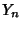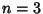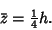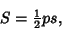## Pyramid

A Polyhedron with one face a Polygon and all the other faces Triangles with a common Vertex. An-gonal regular pyramid (denoted) has Equilateral Triangles, and is possible only for, 4, 5. These correspond to the Tetrahedron, Square Pyramid, and Pentagonal Pyramid, respectively. A pyramid therefore has a single cross-sectional shape in which the length scale of the Cross-Section scales linearly with height. The Area at a heightis given by(1)

whereis the base Area andis the pyramid height. The Volume is therefore given by(2)

These results also hold for the Cone, Tetrahedron (triangular pyramid), Square Pyramid, etc.

The Centroid is the same as for the Cone, given by(3)

The Surface Area of a pyramid is(4)

whereis the Slant Height andis the base Perimeter. Joining two Pyramids together at their bases gives a Bipyramid, also called a Dipyramid.

See also Bipyramid, Elongated Pyramid, Gyroelongated Pyramid, Pentagonal Pyramid, Pyramid, Pyramidal Frustum, Square Pyramid, Tetrahedron, Truncated Square Pyramid

References

Beyer, W. H. (Ed.) CRC Standard Mathematical Tables, 28th ed. Boca Raton, FL: CRC Press, p. 128, 1987.

Hart, G. W. Pyramids, Dipyramids, and Trapezohedra.'' http://www.li.net/~george/virtual-polyhedra/pyramids-info.html.